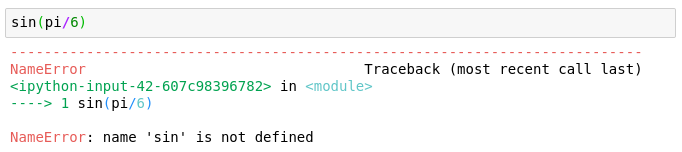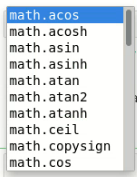# Basic math in Python¶

This notebook works through the use of Python at the level of a calculator, with attention paid to common mathematical operations. We also briefly discuss the use of the math library, variables, types, type conversion, and printing.

Together with this, you should read Chapter 1 of

• Programming for Computations - Python: A Gentle Introduction to Numerical Simulations with Python 3.6 by Svein Linge and Hans Petter Langtangen, 2nd edition.

## Python as a calculator:¶

You can use Python as a calculator to get comfortable working with numbers in Python. Here are some examples.

In :
5+3

Out:
8
In :
35 * 45

Out:
1575
In :
1 / 3

Out:
0.3333333333333333
In :
2 * (3 + 4)

Out:
14

Exponentiation is denoted with the ** operator. (The symbol ^ is reserved for bitwise exclusive or.)

In :
2**3

Out:
8
In :
2^3

Out:
1

The number 52 divided by 3 is 17 with a remainder of 1. Python can compute 17 and 1 with the // and % operators.

In :
52 // 3

Out:
17
In :
52 % 3

Out:
1

Note that a // b always returns the greatest integer less than or equal to $\frac{a}{b}$. This is important for how answers are returned for negative numbers. Also if a // b is $c$ and a % b is $d$, then we have $a = b*c + d$. This explains the following output:

In :
-52 // 3

Out:
-18
In :
-52 % 3

Out:
2
In :
-52 // -3

Out:
17
In :
-52 % -3

Out:
-1

You can also test compare numbers with ==, !=, <, >, <= and >=. These represent the mathematical relations $=$, $\neq$, $<$, $>$, $\leq$ and $\geq$ respectively.

In :
3 == 5

Out:
False
In :
2**3 == 8

Out:
True
In :
2 < 3

Out:
True
In :
4 <= 3

Out:
False
In :
1 != 2 / 2

Out:
False
In :
1/3 == 0.3333

Out:
False
In :
1/3 == 0.333333333333333333333333

Out:
True

## Using the math library¶

The math library contains numerous useful mathematical functions, which are listed in the Python documentation for the math library.

Trigonometry is done in radians. For example, to compute $\cos \frac{\pi}{4}$, you might do the following:

In :
from math import pi, cos
cos(pi/4)

Out:
0.7071067811865476

The line from math import pi, cos allows us to use the pi constant and the cos function from the library. Once this is done, we can continue to use these in the rest of the file.

In :
cos(-2*pi/6)

Out:
0.5000000000000001

Note that we didn't import the sin function so typing sin(pi/6) would result in an error as depicted below:We can solve this problem by importing sin as well.

In :
from math import sin
sin(pi/6)

Out:
0.49999999999999994

## Other types of importing¶

You can import a whole library, as demonstrated below.

In :
import math


When you import the whole library math as above you need to write math.something to access the something in the math library. For example

In :
math.tan(math.pi/4)

Out:
0.9999999999999999
In :
math.e

Out:
2.718281828459045

A good thing about doing this is you can learn about the math library by playing around. For example typing math. followed by pressing the Tab button will lead to a list of objects in the math library. When I do this, I get the following popup list.See if you can also get this list to appear, and scroll through it.

Python also has self contained documentation. For example, you might wonder what the atan2 function does. Typing math.atan2? and pressing Shift+Return produces the documentation. Try it.

In :
math.atan2?


You can also see the documentation of things imported in the other way with something like cos?.

You can also import math with a different name. This is often used to simplify a library name or to avoid name clashes. For example the following imports the math library with the name m and does a simple calculation.

In :
import math as m
m.exp(2)

Out:
7.38905609893065

For more information on importing see §1.4 of Programming for Computations - Python by Svein Linge and Hans Petter Langtangen, 2nd edition.

## Variables¶

Variables can be assigned values with the = operator.

In :
x = 3


The variable keeps the same value until it is changed by the program. For example, the following line prints the value of x.

In :
print(x)

3


This can also be accomplished with the following, because by default Jupyter prints the value produced at the end of a code cell.

In :
x

Out:
3

In math the statement x=x+1 is always False (assuming x is a real number). In Python, it means that the program should first compute the value of x+1 and then store it in the variable x. This results in 4 being stored in x.

In :
x = x+1
print(x)

4


Note that variables can store more than just numbers. Here, we store and add two strings (which concatenates).

In :
s1 = "We are using "
s2 = "Python to compute."
print(s1+s2)

We are using Python to compute.


Here we store a 4-tuple in v.

In :
v = (1,2,3,4)
print(v)

(1, 2, 3, 4)


That v is a tuple can be determined using the type function.

In :
type(v)

Out:
tuple

Also the length (or number of elements) in the tuple can be found using the len function.

In :
len(v)

Out:
4

You can access the individual entries using the form v[i] where i runs from zero up through one less than the number of entries in v. For example the 4th entry can be obtained by:

In :
v

Out:
4

Tuples are not vectors in the sense that algebraic operations mean something different. For example, 3*v is three copies of v concatenated.

In :
3*v

Out:
(1, 2, 3, 4, 1, 2, 3, 4, 1, 2, 3, 4)

Addition concatenates. For example:

In :
v + (5, 6)

Out:
(1, 2, 3, 4, 5, 6)

## Types¶

You have probably noticed that there are at least two different types of numbers: integers and floating point numbers. You can determine the type of a number x with type(x). For example:

In :
a = 3
type(a)

Out:
int
In :
x = 4/3
type(x)

Out:
float
In :
type('Hello!')

Out:
str

Many natural conversions between types are built in functions. For example to convert from an integer to a float, you use:

In :
float(2)

Out:
2.0

You can also go the other way.

In :
int(3.0)

Out:
3

You can use str(x) to convert x to a string. This together with the + operator for string concatenation is a useful way of displaying variable values. For example:

In :
import math
theta = math.pi/5
'tan(' + str(theta) + ') = ' + str(math.tan(theta))

Out:
'tan(0.6283185307179586) = 0.7265425280053609'

## Basic string formatting¶

Using concatenation to form strings makes reading the code difficult. Python has some built in ways to make this process easier and more efficient.

An equivalent to construct the string above line is given below:

In :
'tan({}) = {}'.format(theta, math.tan(theta))

Out:
'tan(0.6283185307179586) = 0.7265425280053609'

The above command works because strings have a format method. Basically, the format method looks for instances of {} and replaces these curly brackets with string representations of arguments passed the the format method. In the above example we have two curly brackets and two parameters (theta and math.tan(theta)). The parameters are converted to strings and used to replace the curly brackets.

The format syntax is pretty powerful, but for now we will be content with basic useage. Section 1.6.2 of LL goes into more detail, including a discussion of formatting numbers.

If you are interested in learning more, concentrate on looking at the examples in the Python documentation of the format string syntax.

Another option for formatting is to use f-strings. We can format the same string with the following:

In :
f'tan({theta}) = {math.tan(theta)}'

Out:
'tan(0.6283185307179586) = 0.7265425280053609'

You can tell this is an f-string because the string has an f in front. The f-string is automatically converted to a string by replacing {theta} with str(theta) and {math.tan(theta)} with str({math.tan(theta)}).

You can learn more about f-strings from PEP 498 -- Literal String Interpolation.

## Multi-line code and printing¶

You might have noticed that Jupyter only prints the results from the last line executed. So for example, 3 is not printed below even though $3 = 1+2$.

In :
1+2
3+4

Out:
7

The simple fix for this is to use the print statement if you want to see an intermediate value:

In :
print(1+2)
3+4

3

Out:
7

Here, 3 is computed as $1+2$, and then converted to a string and displayed by Jupyter.

This sort of printing is very useful for debugging. For example, below we have attempted an implementation of the quadratic formula to find the roots of $$(2 x-1)(x-2)=2 x^2 - 5x +2.$$ (From the factorization above, you can see that the roots should be $1/2$ and $2$.)

In :
a = 2  # coefficient of x^2
b = -5 # coefficient of x
c = 2  # constant coefficient
q = math.sqrt(b*b - 4*a*c)
root1 = (-b + q)/2*a
root2 = (-b - q)/2*a
print(f'The roots are {root1} and {root2}.')

The roots are 8.0 and 2.0.


So, there is some error in our code. To debug you might check that the value of $q = \sqrt{b^2-4ac}$ is correct. In our example this should be $\sqrt{25-16}=3.$ Let's check our code:

In :
a = 2  # coefficient of x^2
b = -5 # coefficient of x
c = 2  # constant coefficient
q = math.sqrt(b*b - 4*a*c)
print(f'q = {q}')
root1 = (-b + q)/2*a
root2 = (-b - q)/2*a
print(f'The roots are {root1} and {root2}.')

q = 3.0
The roots are 8.0 and 2.0.


Well the value of $q$ is correct, so the problem must be in the formulas for root1 and root2. Ah... Multiplication and division have the same precidence and so the code is computing $-b+q$, dividing it by $2$ and then multiplying the result by $a$. We really want to divide by $2a$. So, a fix is below:

In :
a = 2  # coefficient of x^2
b = -5 # coefficient of x
c = 2  # constant coefficient
q = math.sqrt(b*b - 4*a*c)
print(f'q = {q}')
root1 = (-b + q)/(2*a)
root2 = (-b - q)/(2*a)
print(f'The roots are {root1} and {root2}.')

q = 3.0
The roots are 2.0 and 0.5.


Anyway, the point I want to make is that printing intermediate values can allow you to zoom in on the problematic lines in code with errors.